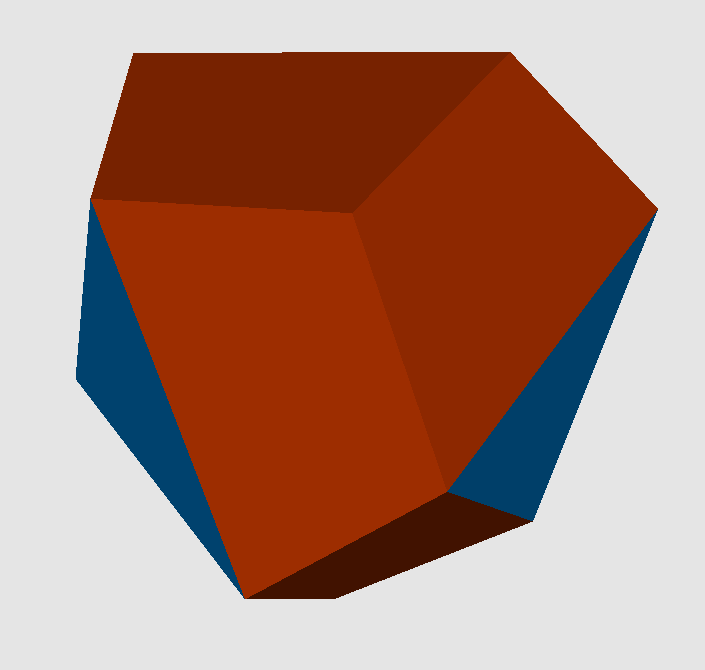Acronym ... Name tetrahedrally diminished dodecahedron,vertex figure of pd{3,5,3} ```© ``` Circumradius sqrt[(9+3 sqrt(5))/8] = 1.401259 Vertex figure [3,t,T,t], [T3] Dihedral angles (at margins) between {3} and {(t,t,T,T)}:   arccos(-sqrt[(5+2 sqrt(5))/15]) = 142.622632° between {(t,t,T,T)} and {(t,t,T,T)}:   arccos(-1/sqrt(5)) = 116.565051° Confer doe   tet   cube-dim-doe

The non-regular (fxxx)-trapezia {(t,t,T,T)} are faceted regular pentagons. Its vertex angles are t = 72° resp. T = 108°. Their longer side is scaled by the golden ratio f = (1+sqrt(5))/2 = 1.618034. The triangles are regular, but use the f-scaled edges only.

This polyhedron is chiral and automorph.

Incidence matrix

```12 * |  2 1  1 |  3 1  [3,t,T,t]
* 4 |  0 0  3 |  3 0  [T3]
-----+---------+-----
2 0 | 12 *  * |  1 1  f-edges
2 0 |  * 6  * |  2 0
1 1 |  * * 12 |  2 0
-----+---------+-----
3 1 |  1 1  2 | 12 *  (fxxx)-trapezium
3 0 |  3 0  0 |  * 4  f-{3}
```